Скачать презентацию If a ship s tank is partially filled

Chapter 7 Free surface.ppt

• Количество слайдов: 30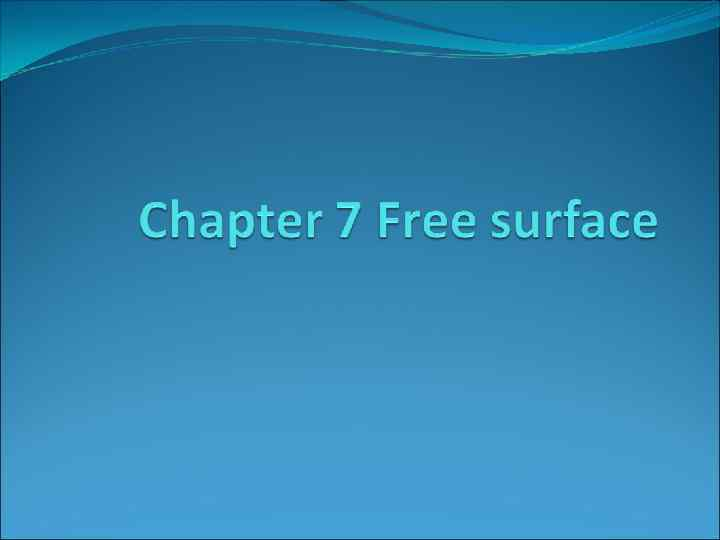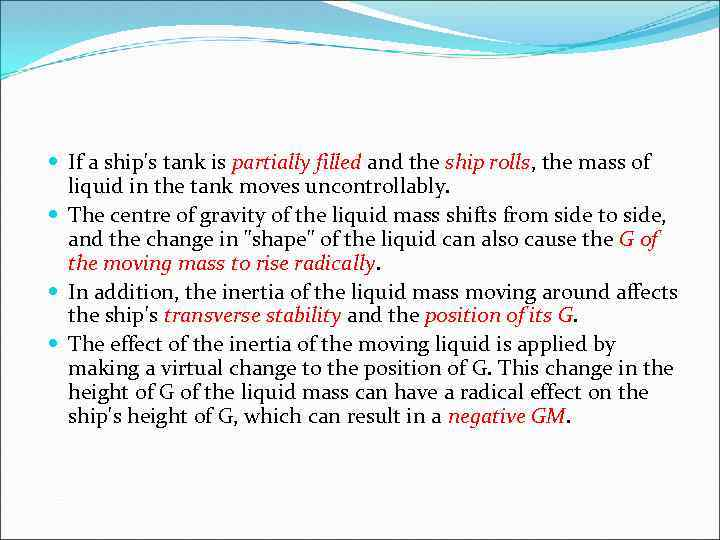If a ship's tank is partially filled and the ship rolls, the mass of liquid in the tank moves uncontrollably. The centre of gravity of the liquid mass shifts from side to side, and the change in "shape" of the liquid can also cause the G of the moving mass to rise radically. In addition, the inertia of the liquid mass moving around affects the ship's transverse stability and the position of its G. The effect of the inertia of the moving liquid is applied by making a virtual change to the position of G. This change in the height of G of the liquid mass can have a radical effect on the ship's height of G, which can result in a negative GM.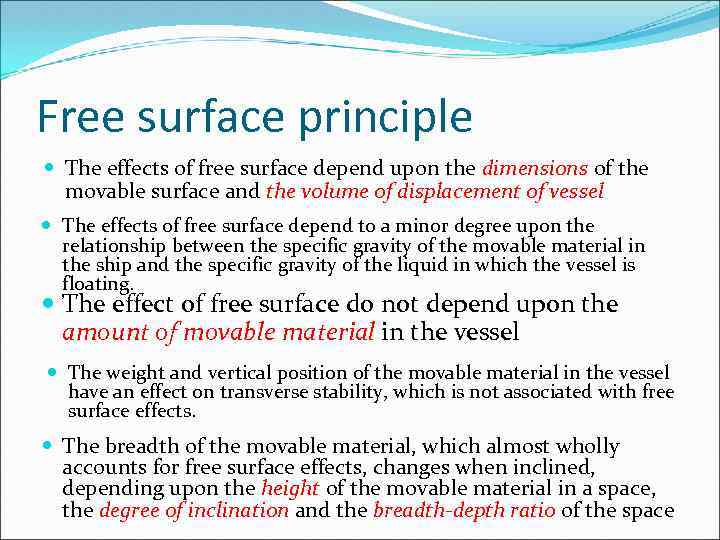Free surface principle The effects of free surface depend upon the dimensions of the movable surface and the volume of displacement of vessel The effects of free surface depend to a minor degree upon the relationship between the specific gravity of the movable material in the ship and the specific gravity of the liquid in which the vessel is floating. The effect of free surface do not depend upon the amount of movable material in the vessel The weight and vertical position of the movable material in the vessel have an effect on transverse stability, which is not associated with free surface effects. The breadth of the movable material, which almost wholly accounts for free surface effects, changes when inclined, depending upon the height of the movable material in a space, the degree of inclination and the breadth-depth ratio of the space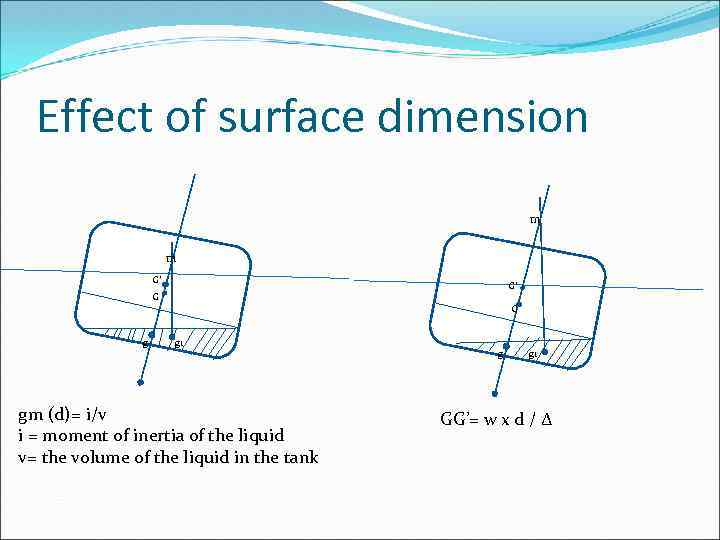Effect of surface dimension m m G’ G’ G g 1 gm (d)= i/v i = moment of inertia of the liquid v= the volume of the liquid in the tank g g 1 GG’= w x d / ∆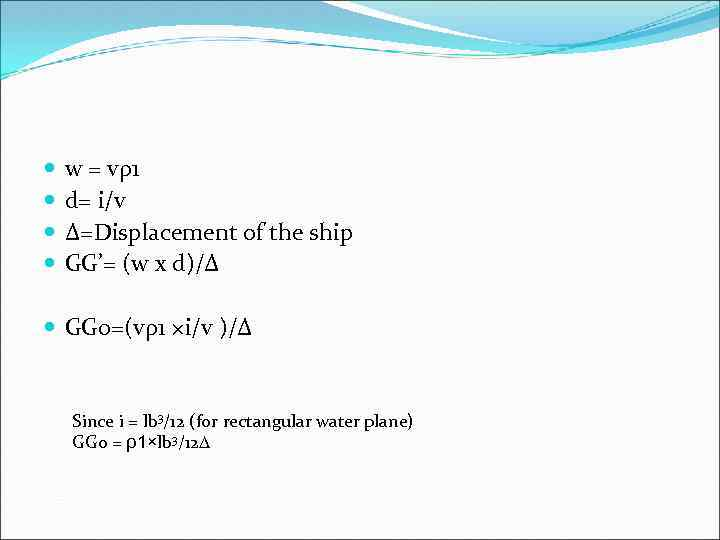w = vρ1 d= i/v ∆=Displacement of the ship GG’= (w x d)/∆ GG 0=(vρ1 ×i/v )/∆ Since i = lb 3/12 (for rectangular water plane) GG 0 = ρ1×lb 3/12∆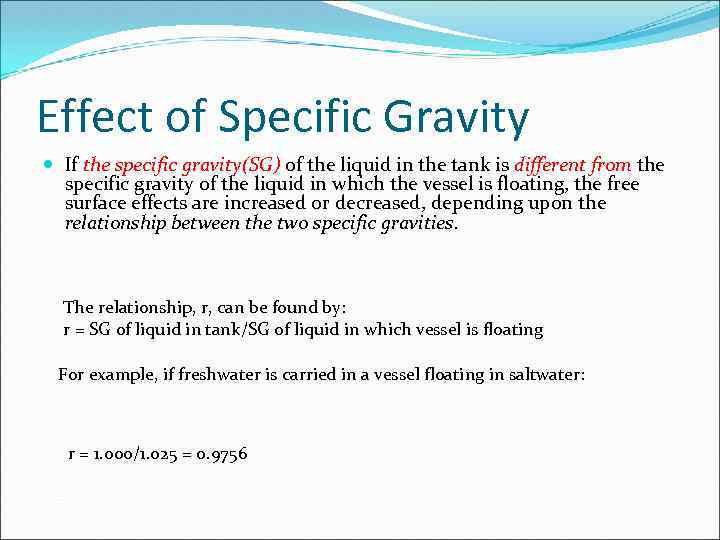Effect of Specific Gravity If the specific gravity(SG) of the liquid in the tank is different from the specific gravity of the liquid in which the vessel is floating, the free surface effects are increased or decreased, depending upon the relationship between the two specific gravities. The relationship, r, can be found by: r = SG of liquid in tank/SG of liquid in which vessel is floating For example, if freshwater is carried in a vessel floating in saltwater: r = 1. 000/1. 025 = 0. 9756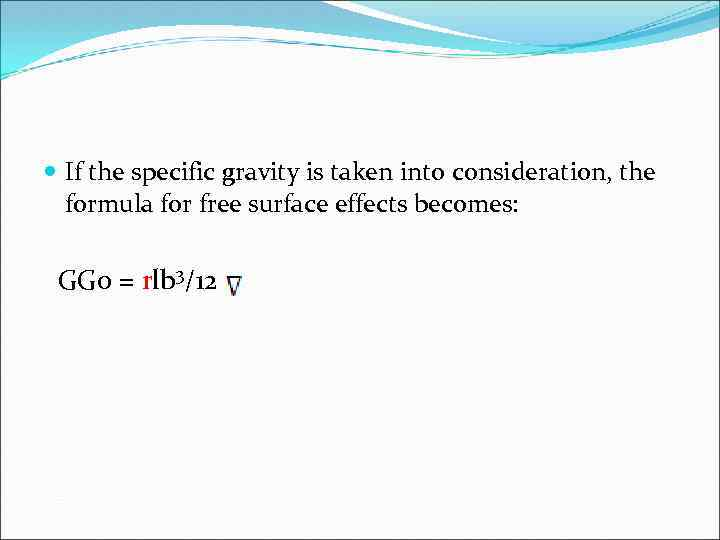If the specific gravity is taken into consideration, the formula for free surface effects becomes: GG 0 = rlb 3/12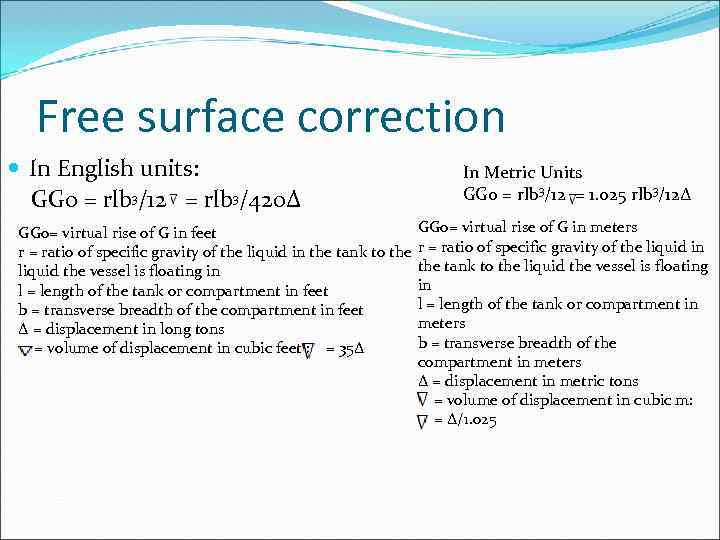Free surface correction In English units: GG 0 = rlb 3/12 = rlb 3/420∆ GG 0= virtual rise of G in feet r = ratio of specific gravity of the liquid in the tank to the liquid the vessel is floating in l = length of the tank or compartment in feet b = transverse breadth of the compartment in feet ∆ = displacement in long tons = volume of displacement in cubic feet : = 35∆ In Metric Units GG 0 = rlb 3/12 = 1. 025 rlb 3/12∆ GG 0= virtual rise of G in meters r = ratio of specific gravity of the liquid in the tank to the liquid the vessel is floating in l = length of the tank or compartment in meters b = transverse breadth of the compartment in meters ∆ = displacement in metric tons = volume of displacement in cubic m: = ∆/1. 025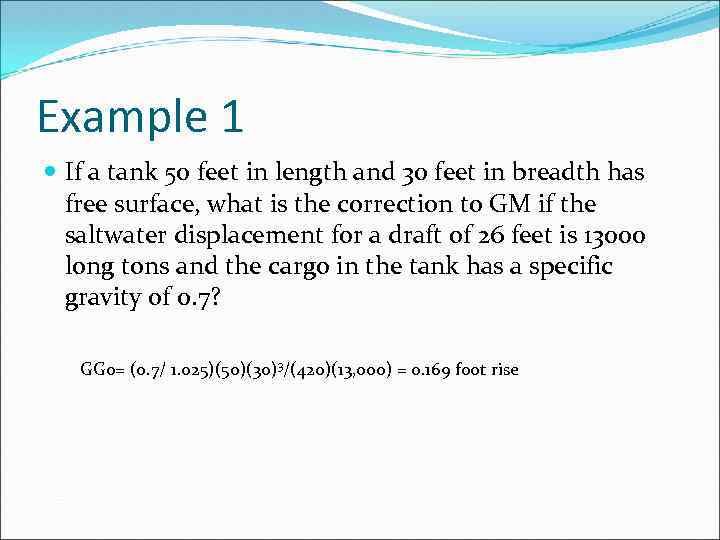Example 1 If a tank 50 feet in length and 30 feet in breadth has free surface, what is the correction to GM if the saltwater displacement for a draft of 26 feet is 13000 long tons and the cargo in the tank has a specific gravity of 0. 7? GG 0= (0. 7/ 1. 025)(50)(30)3/(420)(13, 000) = 0. 169 foot rise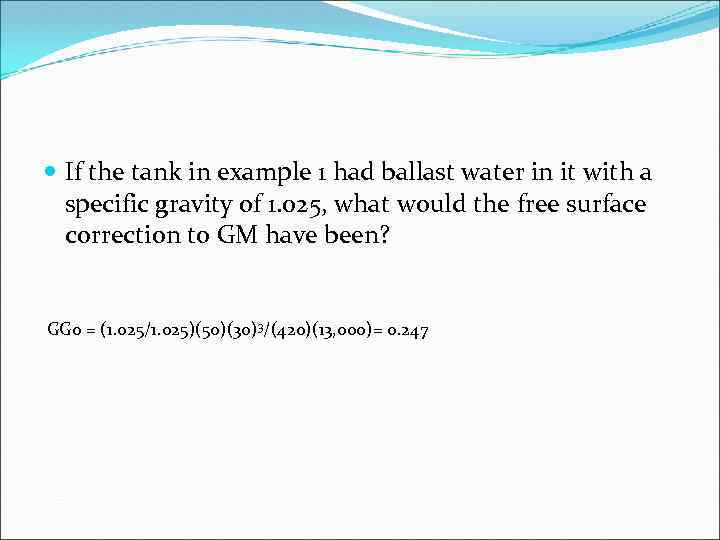If the tank in example 1 had ballast water in it with a specific gravity of 1. 025, what would the free surface correction to GM have been? GG 0 = (1. 025/1. 025)(50)(30)3/(420)(13, 000)= 0. 247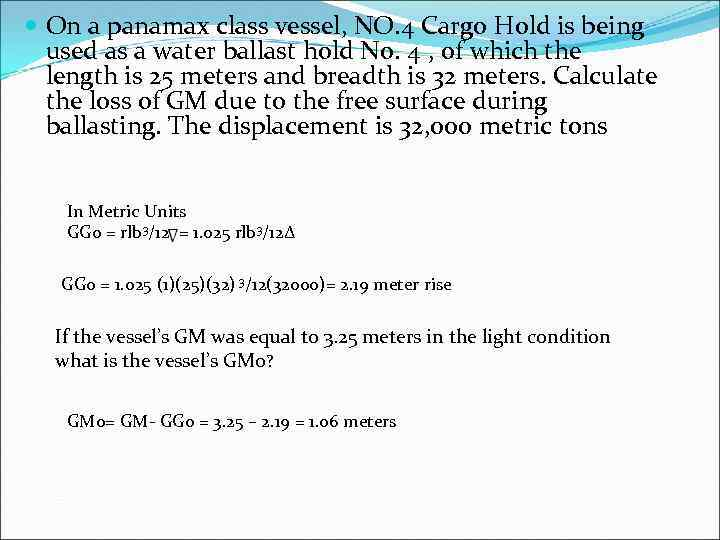On a panamax class vessel, NO. 4 Cargo Hold is being used as a water ballast hold No. 4 , of which the length is 25 meters and breadth is 32 meters. Calculate the loss of GM due to the free surface during ballasting. The displacement is 32, 000 metric tons In Metric Units GG 0 = rlb 3/12 = 1. 025 rlb 3/12∆ GG 0 = 1. 025 (1)(25)(32) 3/12(32000)= 2. 19 meter rise If the vessel’s GM was equal to 3. 25 meters in the light condition what is the vessel’s GM 0? GM 0= GM- GG 0 = 3. 25 – 2. 19 = 1. 06 meters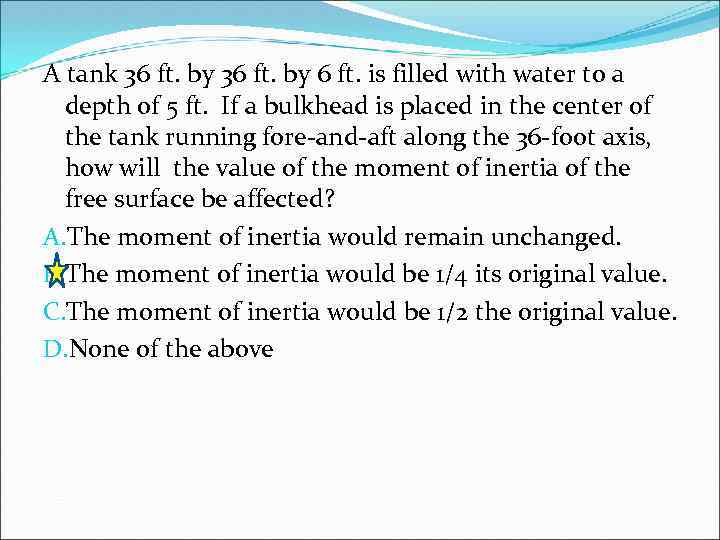A tank 36 ft. by 6 ft. is filled with water to a depth of 5 ft. If a bulkhead is placed in the center of the tank running fore-and-aft along the 36 -foot axis, how will the value of the moment of inertia of the free surface be affected? A. The moment of inertia would remain unchanged. B. The moment of inertia would be 1/4 its original value. C. The moment of inertia would be 1/2 the original value. D. None of the above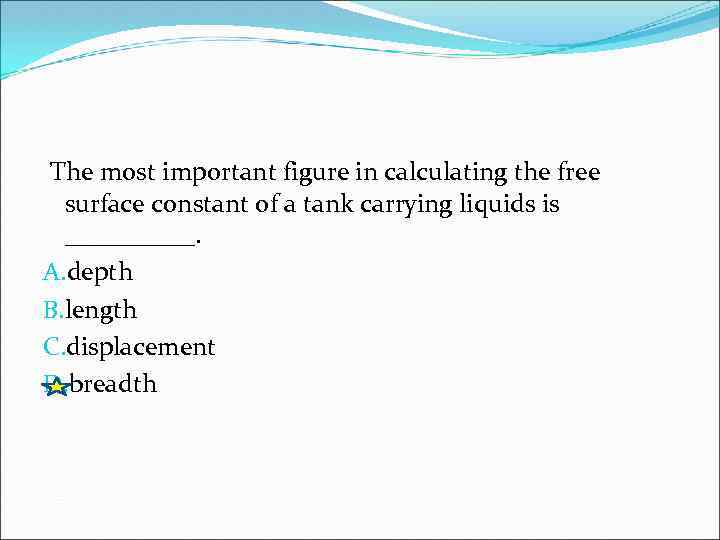The most important figure in calculating the free surface constant of a tank carrying liquids is _____. A. depth B. length C. displacement D. breadth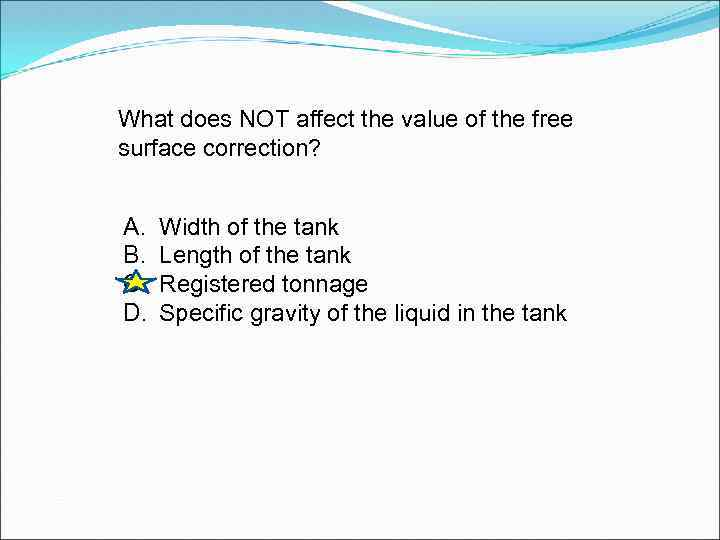What does NOT affect the value of the free surface correction? A. B. C. D. Width of the tank Length of the tank Registered tonnage Specific gravity of the liquid in the tank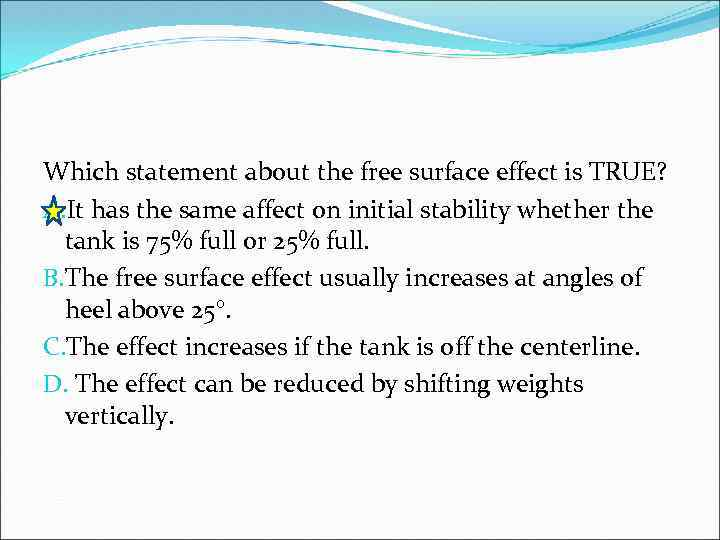Which statement about the free surface effect is TRUE? A. It has the same affect on initial stability whether the tank is 75% full or 25% full. B. The free surface effect usually increases at angles of heel above 25°. C. The effect increases if the tank is off the centerline. D. The effect can be reduced by shifting weights vertically.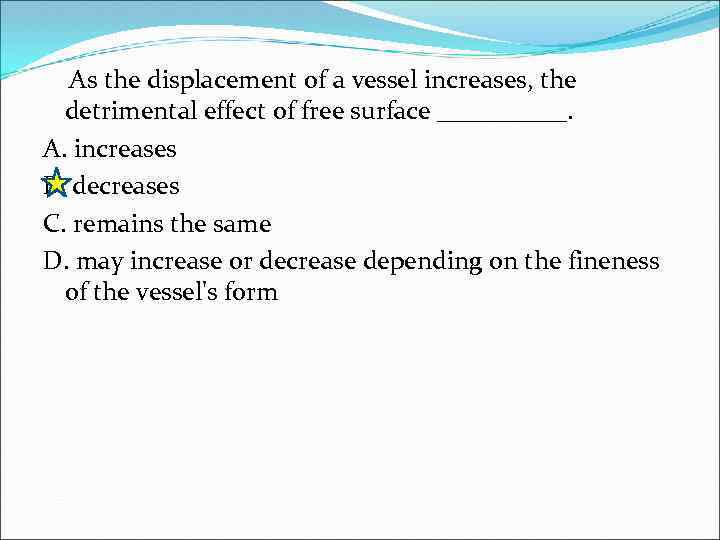As the displacement of a vessel increases, the detrimental effect of free surface _____. A. increases B. decreases C. remains the same D. may increase or decrease depending on the fineness of the vessel's form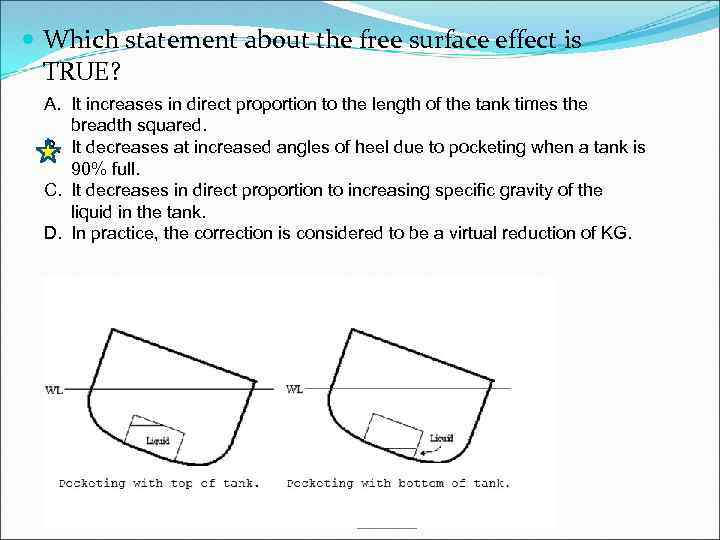Which statement about the free surface effect is TRUE? A. It increases in direct proportion to the length of the tank times the breadth squared. B. It decreases at increased angles of heel due to pocketing when a tank is 90% full. C. It decreases in direct proportion to increasing specific gravity of the liquid in the tank. D. In practice, the correction is considered to be a virtual reduction of KG.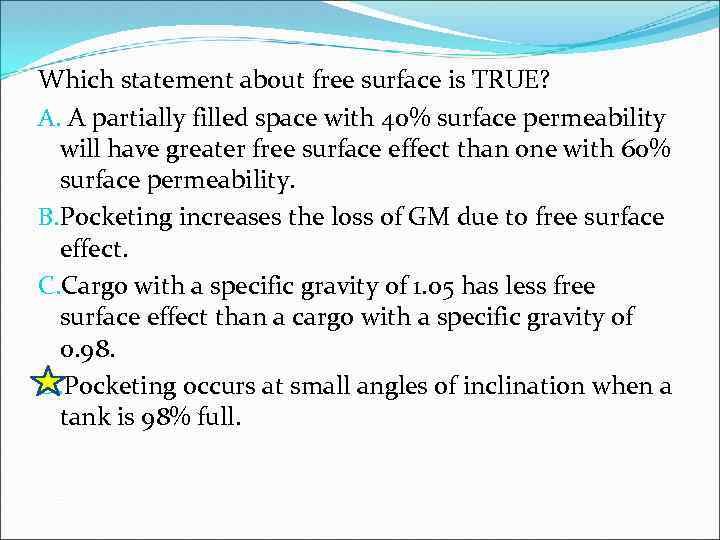Which statement about free surface is TRUE? A. A partially filled space with 40% surface permeability will have greater free surface effect than one with 60% surface permeability. B. Pocketing increases the loss of GM due to free surface effect. C. Cargo with a specific gravity of 1. 05 has less free surface effect than a cargo with a specific gravity of 0. 98. D. Pocketing occurs at small angles of inclination when a tank is 98% full.Which statement about the free surface correction is TRUE? A. It is added to GM at light drafts and subtracted at deep drafts. B. It is increased if the slack tank is not on the centerline. C. It is decreased if the slack tank is below the KG of the vessel. D. The correction decreases as the draft increases due to loading dry cargo.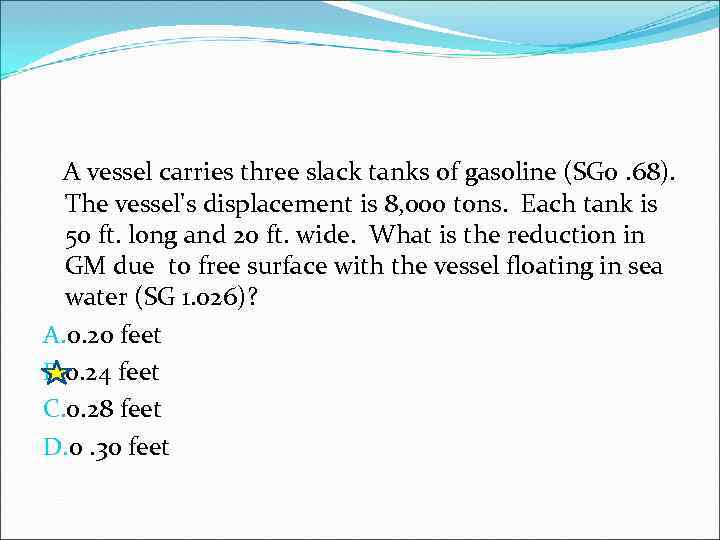A vessel carries three slack tanks of gasoline (SG 0. 68). The vessel's displacement is 8, 000 tons. Each tank is 50 ft. long and 20 ft. wide. What is the reduction in GM due to free surface with the vessel floating in sea water (SG 1. 026)? A. 0. 20 feet B. 0. 24 feet C. 0. 28 feet D. 0. 30 feet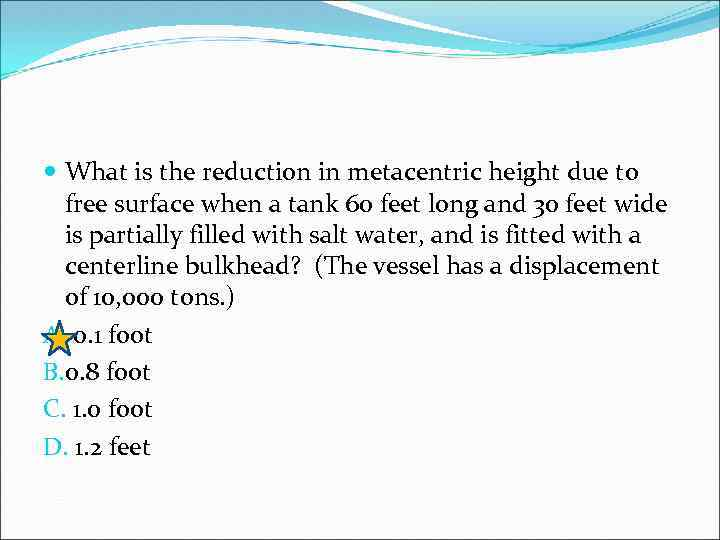What is the reduction in metacentric height due to free surface when a tank 60 feet long and 30 feet wide is partially filled with salt water, and is fitted with a centerline bulkhead? (The vessel has a displacement of 10, 000 tons. ) A. 0. 1 foot B. 0. 8 foot C. 1. 0 foot D. 1. 2 feet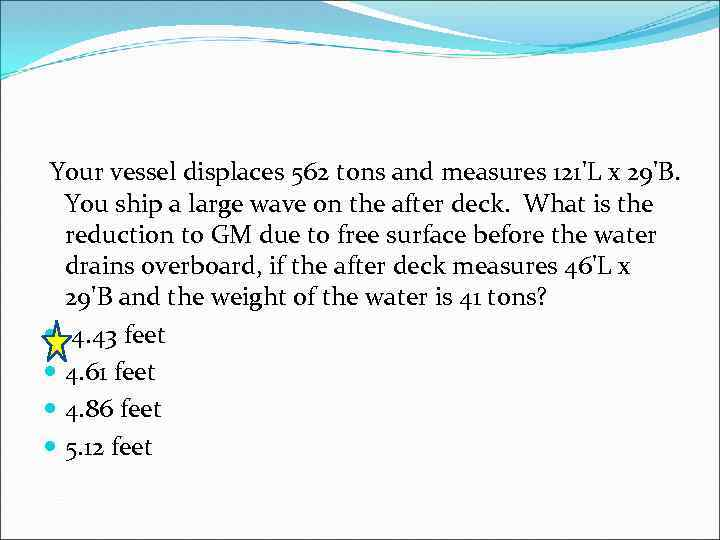Your vessel displaces 562 tons and measures 121'L x 29'B. You ship a large wave on the after deck. What is the reduction to GM due to free surface before the water drains overboard, if the after deck measures 46'L x 29'B and the weight of the water is 41 tons? 4. 43 feet 4. 61 feet 4. 86 feet 5. 12 feet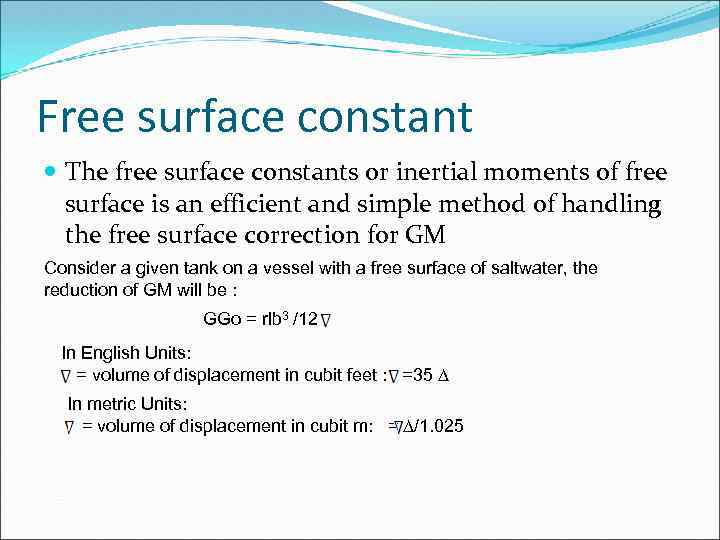Free surface constant The free surface constants or inertial moments of free surface is an efficient and simple method of handling the free surface correction for GM Consider a given tank on a vessel with a free surface of saltwater, the reduction of GM will be : GGo = rlb 3 /12 In English Units: = volume of displacement in cubit feet : =35 ∆ In metric Units: = volume of displacement in cubit m: = ∆/1. 025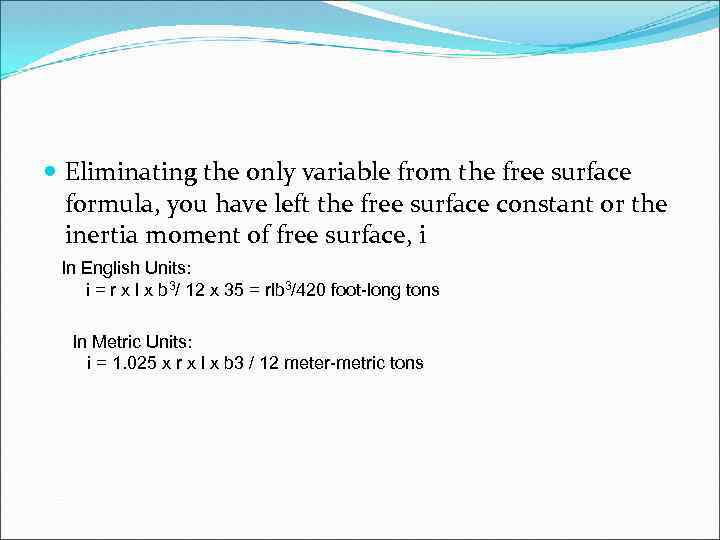Eliminating the only variable from the free surface formula, you have left the free surface constant or the inertia moment of free surface, i In English Units: i = r x l x b 3/ 12 x 35 = rlb 3/420 foot-long tons In Metric Units: i = 1. 025 x r x l x b 3 / 12 meter-metric tons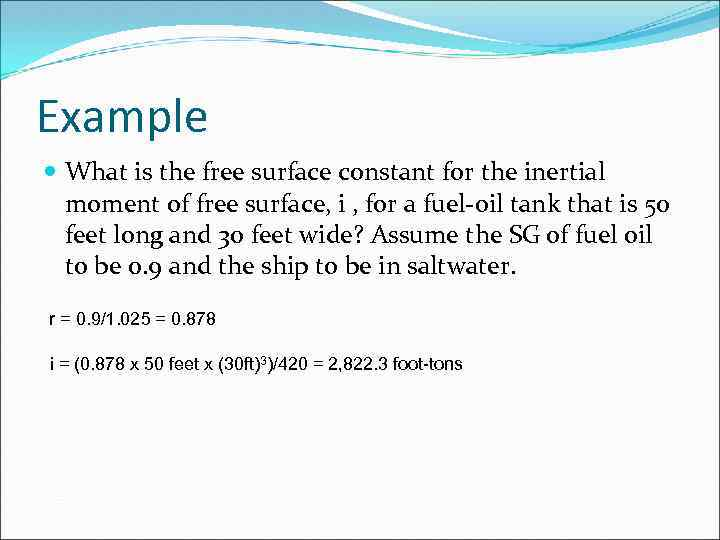Example What is the free surface constant for the inertial moment of free surface, i , for a fuel-oil tank that is 50 feet long and 30 feet wide? Assume the SG of fuel oil to be 0. 9 and the ship to be in saltwater. r = 0. 9/1. 025 = 0. 878 i = (0. 878 x 50 feet x (30 ft)3)/420 = 2, 822. 3 foot-tons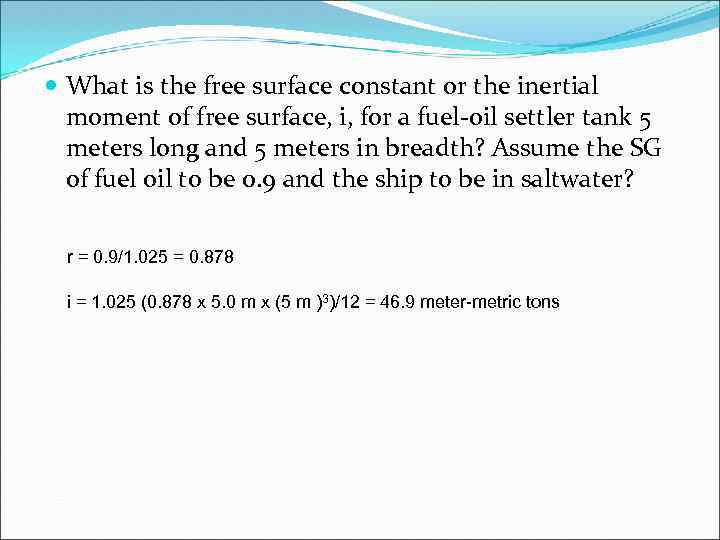What is the free surface constant or the inertial moment of free surface, i, for a fuel-oil settler tank 5 meters long and 5 meters in breadth? Assume the SG of fuel oil to be 0. 9 and the ship to be in saltwater? r = 0. 9/1. 025 = 0. 878 i = 1. 025 (0. 878 x 5. 0 m x (5 m )3)/12 = 46. 9 meter-metric tons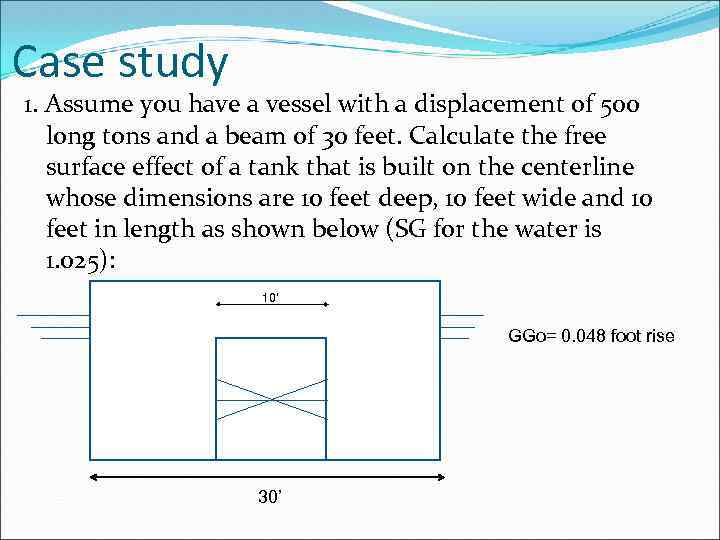Case study 1. Assume you have a vessel with a displacement of 500 long tons and a beam of 30 feet. Calculate the free surface effect of a tank that is built on the centerline whose dimensions are 10 feet deep, 10 feet wide and 10 feet in length as shown below (SG for the water is 1. 025): 10’ GGo= 0. 048 foot rise 30’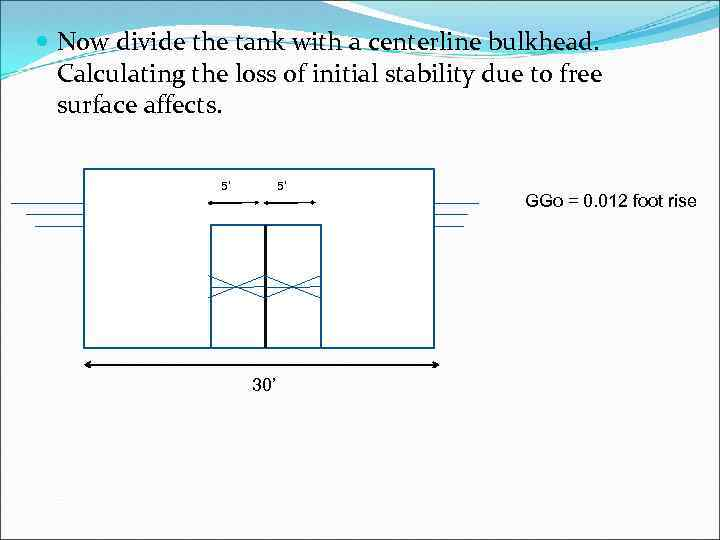Now divide the tank with a centerline bulkhead. Calculating the loss of initial stability due to free surface affects. 5’ 5’ 30’ GGo = 0. 012 foot rise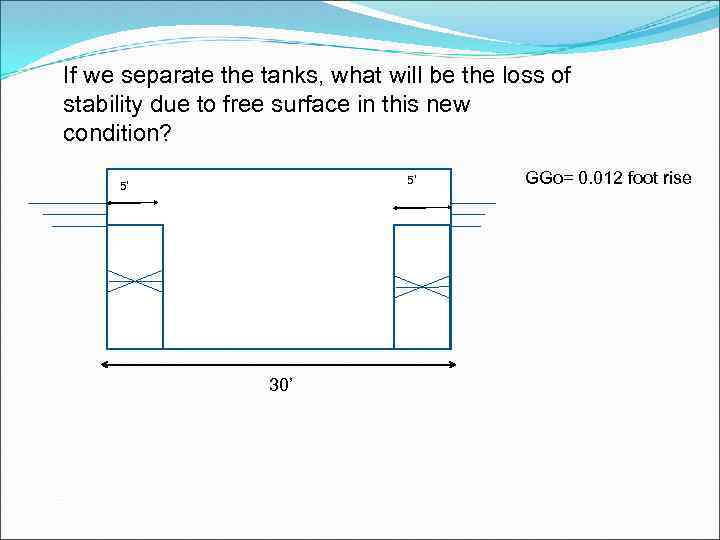If we separate the tanks, what will be the loss of stability due to free surface in this new condition? 5’ 5’ 30’ GGo= 0. 012 foot rise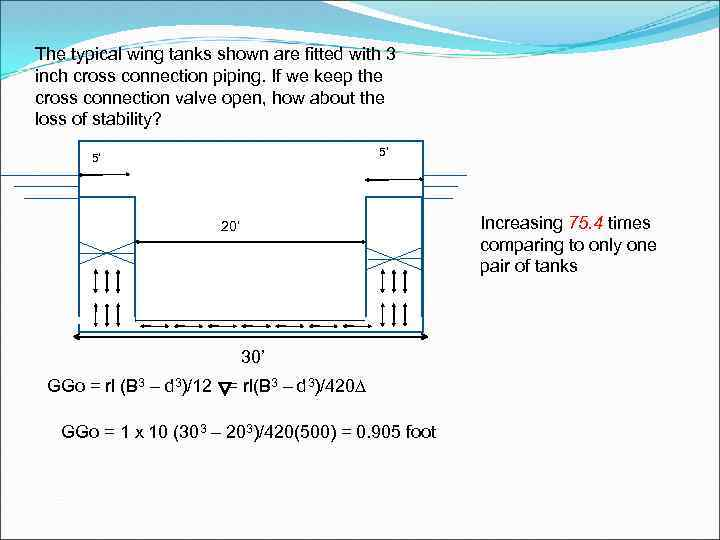The typical wing tanks shown are fitted with 3 inch cross connection piping. If we keep the cross connection valve open, how about the loss of stability? 5’ 5’ Increasing 75. 4 times comparing to only one pair of tanks 20’ 30’ GGo = rl (B 3 – d 3)/12 = rl(B 3 – d 3)/420∆ GGo = 1 x 10 (303 – 203)/420(500) = 0. 905 foot